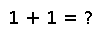# get_zero_subnormals

get_zero_subnormals() -> Bool

Returns `false` if operations on subnormal floating-point values ("denormals") obey rules for IEEE arithmetic, and `true` if they might be converted to zeros.

## Examples

Checking you are not a robot: